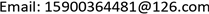CSAComputer Science and Application2161-8801Scientific Research Publishing10.12677/CSA.2020.109162CSA-37430CSA20200900000_67676549.pdf信息通讯 用于无人机巡线的图像分类模型选择算法研究 Research on Algorithm of Image Classification Model Selection for UAV Patrol 明磊2121文璇31国家海洋技术中心，天津天津航天中为数据系统科技有限公司，天津null02092020100915411548© Copyright 2014 by authors and Scientific Research Publishing Inc. 2014This work is licensed under the Creative Commons Attribution International License (CC BY). http://creativecommons.org/licenses/by/4.0/

Research on Algorithm of Image Classification Model Selection for UAV Patrol

Minglei Jin1, Ming Li1, Wenxuan Zhao2

1Tianjin Zhongwei Aerospace Data System Technology Co. Ltd., Tianjin

2National Ocean Technology Center, TianjinReceived: Aug. 15th, 2020; accepted: Aug. 26th, 2020; published: Sep. 2nd, 2020ABSTRACT

As a classic classification algorithm, random forest algorithm is widely used and has high classification accuracy. However, in the process of classification, the classification performance of each decision tree and the difference between two decision trees are two important factors that affect the final classification effect. When some decision trees have similar misclassifications, and they are used in the final voting on the results of the decision tree, the final classification effect of the model will be reduced. Aiming at this problem, this paper proposes a method for measuring the similarity of decision trees based on confusion Matrix. This method takes into account the number of different categories of trees and the correct and incorrect classification, in order to select decision trees with weak similarity, and then remove the decision trees with poor classification results, and finally complete the model selection of random forest. Experimental results show that the method proposed in this paper has a higher average classification accuracy rate and higher stability in the three types of datasets.

Keywords:Integrated Classifier, Random Forest, Confusion Matrix

Copyright © 2020 by author(s) and Hans Publishers Inc.

This work is licensed under the Creative Commons Attribution International License (CC BY 4.0).

http://creativecommons.org/licenses/by/4.0/1. 引言

2. 集成学习集成分类器的差异性度量

E t o t a l = E b a y + 1 + ρ ( N − 1 ) N E e a c h (1)

3. 随机森林算法

1) 从训练集中采用bootstrap法，即自助抽样法，有放回地抽取若干样本，作为一个训练子集。

2) 对于训练子集，从特征集中无放回地随机抽取若干特征，作为决策树的每个节点的分裂的依据。

3) 重复步骤1)和步骤2)，得到若干训练子集，并生产若干决策树，将决策树组合起来，形成随机森林。

4) 将测试集的样本输入随机森林中，让每个决策树对样本进行决策，得到结果后，采用投票方法对结果投票，得到样本的分类结果。

5) 重复步骤4)，直到测试集分类完成。

4. 随机森林模型选择4.1. 本文提出的模型选择方法

4.1.1. 基于误差矩阵判断分类树相似性

C N = [ c n 11 ⋯ c n 1 m ⋮ c n i i ⋮ c n m 1 ... c n m m ] (2)

D C N ( i , j ) = C N ( i ) − C N ( j ) = [ c n 11 ( i ) − c n 11 ( j ) c n 12 ( i ) − c n 12 ( j ) ⋯ c n 1 N ( i ) − c n 1 M ( j ) c n 21 ( i ) − c n 21 ( j ) c n 22 ( i ) − c n 22 ( j ) ⋯ c n 2 N ( i ) − c n 2 M ( j ) ⋮ ⋮ ⋱ ⋮ c n M 1 ( i ) − c n M 1 ( j ) c n M 2 ( i ) − c n M 2 ( j ) ⋯ c n M M ( i ) − c n M M ( j ) ] (3)

d c n ′ m n = d c n m n m a x m (4)

m a x m = m a x n ( d c n m n ) (5)

r f i j = { 0 ,     ( i ≥ j ) ‖ D C N ′ i , j ‖ F = ∑ m M ∑ n M d c n ′ m n 2 ,     ( i < j ) (6)

4.1.2. 基于“删劣”策略选择模型

4.1.3. 模型选择算法描述

1：通过决策树对测试样本进行分类预测；

2：根据分类结果，为决策树创建误差矩阵 C N

3：创建相似度度量矩阵 R F ：

4：for (( i , j = 1 to l) & ( i < j ))

5：令 m i j 为 R F 中最小的非零元素

6：for ( m i j < t )

if (决策树i分类效果 < β )

m i j = R F 中下一个最小非零元素

7：否则结束，未删除的决策树组成随机森林RF。

5. 实验与分析5.1. 实验数据说明

Experimental data set taken from UC
UCI数据集属性类别特征维数样本个数类别个数
Iris连续41503
Breast-cancer离散102862
Anneal离散连续387988

5.2. 实验结果与分析

6. 结束语